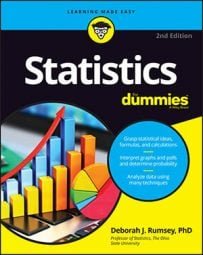##### Statistics For DummiesWhen working with statistics, it’s important to recognize the different types of data: numerical (discrete and continuous), categorical, and ordinal.

Data are the actual pieces of information that you collect through your study. For example, if you ask five of your friends how many pets they own, they might give you the following data: 0, 2, 1, 4, 18. (The fifth friend might count each of their aquarium fish as a separate pet — and who are we to take that from them?) Not all data are numbers; let’s say you also record the gender of each of your friends, getting the following data: male, male, female, male, female.

Most data fall into one of two groups: numerical or categorical.

## Numerical data

These data have meaning as a measurement, such as a person’s height, weight, IQ, or blood pressure; or they’re a count, such as the number of stock shares a person owns, how many teeth a dog has, or how many pages you can read of your favorite book before you fall asleep. (Statisticians also call numerical data quantitative data.)

Numerical data can be further broken into two types: discrete and continuous.

• Discrete data represent items that can be counted; they take on possible values that can be listed out. The list of possible values may be fixed (also called finite); or it may go from 0, 1, 2, on to infinity (making it countably infinite).For example, the number of heads in 100 coin flips takes on values from 0 through 100 (finite case), but the number of flips needed to get 100 heads takes on values from 100 (the fastest scenario) on up to infinity (if you never get to that 100th heads). Its possible values are listed as 100, 101, 102, 103 . . . (representing the countably infinite case).
• Continuous data represent measurements; their possible values cannot be counted and can only be described using intervals on the real number line. For example, the exact amount of gas purchased at the pump for cars with 20-gallon tanks would be continuous data from 0 gallons to 20 gallons, represented by the interval [0, 20], inclusive. You might pump 8.40 gallons, or 8.41, or 8.414863 gallons, or any possible number from 0 to 20. In this way, continuous data can be thought of as being uncountably infinite. For ease of recordkeeping, statisticians usually pick some point in the number to round off. Another example would be that the lifetime of a C battery can be anywhere from 0 hours to an infinite number of hours (if it lasts forever), technically, with all possible values in between. Granted, you don’t expect a battery to last more than a few hundred hours, but no one can put a cap on how long it can go (remember the Energizer Bunny?).

## Categorical data

Categorical data represent characteristics such as a person’s gender, marital status, hometown, or the types of movies they like. Categorical data can take on numerical values (such as “1” indicating male and “2” indicating female), but those numbers don’t have mathematical meaning. You couldn’t add them together, for example. (Other names for categorical data are qualitative data, or Yes/No data.)

## Ordinal data

Ordinal data mixes numerical and categorical data. The data fall into categories, but the numbers placed on the categories have meaning. For example, rating a restaurant on a scale from 0 (lowest) to 4 (highest) stars gives ordinal data.

Ordinal data are often treated as categorical, where the groups are ordered when graphs and charts are made. However, unlike categorical data, the numbers do have mathematical meaning. For example, if you survey 100 people and ask them to rate a restaurant on a scale from 0 to 4, taking the average of the 100 responses will have meaning. This would not be the case with categorical data.